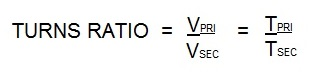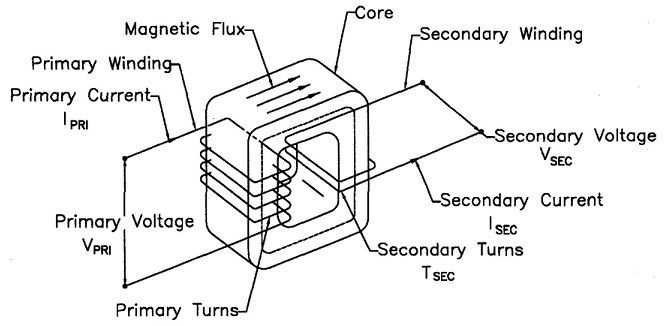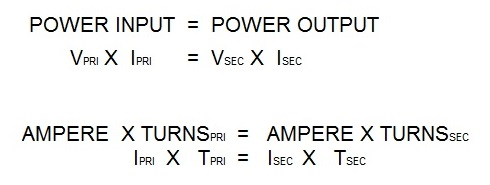# What is the turns ratio for a 16 kva resistance welding transformer?

The turns ratio for all resistance welding transformers is calculated in the same manner no matter the KVA size. Turns ratio is the ratio of the number of coil turns in the primary vs the secondary.In AC transformers, there are many turns in the primary. The secondary has one turn. If there are 50 turns then the ratio is 50/1. Therefor the primary voltage will drop from 220 V and 100 amp input to 220/50 = 4.4 volts in the secondary. The equations for this are:Vpri = Voltage on the primary                   Tpri = Turns in the primary coil

Vsec= Voltage on the secondary              Tsec = Turns in the secondary coilPower into the transformer will equal power out of the transformer.Isec = Secondary amperage

Ipri = Primary amperage

In the secondary using the above formula:

If the Ipri = 100 amperes

If the turns ratio = 50/1

Isec = (Ipri X Tpri)/ Tsec

Isec = 100 x 50/1

Isec = 5000 amperes

There are several additional articles written on turns ratio available in the “HOW TO RESISTANCE WELD” website. Enter “Turns Ratio” in the search box on the home page for additional information.

Reference: RWMA Manual Chapter 19

### Have a Question?

Do you have a question that is not covered in our knowledgebase? Do you have questions regarding the above article? Click here to ask the professor.